October 17, 2019

## What is a linear model?

A statistical model where a response is estimated as a linear function of predictors.

Linear operations include:

• Multiplying vectors by a constant (*)

Simplest case:

• $$y = mx + b$$ (grade school)
• $$Y_i = \beta_0 + \beta_1 X_i + \epsilon_i$$ (grown-up)

An observation (i) has a mean value that is estimated by an intercept coefficient ($$\beta_0$$) and a slope coefficient ($$\beta_1$$) multiplied by a predictor ($$X$$) with some random error ($$\epsilon_i$$)

## Variations

Case 1: Classic linear model | lm()

• Observations (Y’s) come from a normal distribution with constant variance
• Predictors are fixed (not random)

Case 2: Random / Mixed Effects Model | lmer(), lme()

• Observations (Y’s) come from a normal distribution with constant variance
• Predictors can be either fixed OR random

Case 3: Generalized Linear Model | glm(), glmer()

• Observations (Y’s) can come from a distribution whose mean is estimated by a linear function of predictors.
• Poisson regression, logistic regression

Despite differences in linear model types, analysis steps and model formulation are remarkably consistent

Here, we’ll be using data from the mtcars (Motor Trends Car Road Tests) dataset to explore the basics of linear modeling.

cars_subset <- mtcars %>% select(mpg, disp, hp, wt, drat)

1. Define question
2. Collect and clean data
3. Exploring predictors
4. Fitting model objects
5. Model diagnostics
6. Inference and visualization

Have a defined question (or hypothesis) when starting analysis can help guide your modeling approach.

• What predictor variables are of interest?
• Am I looking to test the fit of a particular model?
• Do I want to compare many potential models?
• Am I interested in finding the combination of variables that best predicts a response?

As an example:

What variables best predict the fuel efficiency (mpg) of a car?

Keep this question in mind at all times going forward

## 2. Collect and clean data

Will not cover this in much detail today, but know that:

Data must be in a consistent for many modeling packages

• Rows correspond to unique observations
• Columns correspond to variables (dependent and independent)
##                    mpg disp  hp    wt drat
## Mazda RX4         21.0  160 110 2.620 3.90
## Mazda RX4 Wag     21.0  160 110 2.875 3.90
## Datsun 710        22.8  108  93 2.320 3.85
## Hornet 4 Drive    21.4  258 110 3.215 3.08
## Hornet Sportabout 18.7  360 175 3.440 3.15
## Valiant           18.1  225 105 3.460 2.76

## 3. Exploring predictors

How are my predictors distributed?

• Highly skewed predictors can be problematic – outlying points and non-linear relationships
• Highly correlated predictors will increase parameter variance

Inspect predictors with hist() or ggplot2::geom_histogram(), transform if needed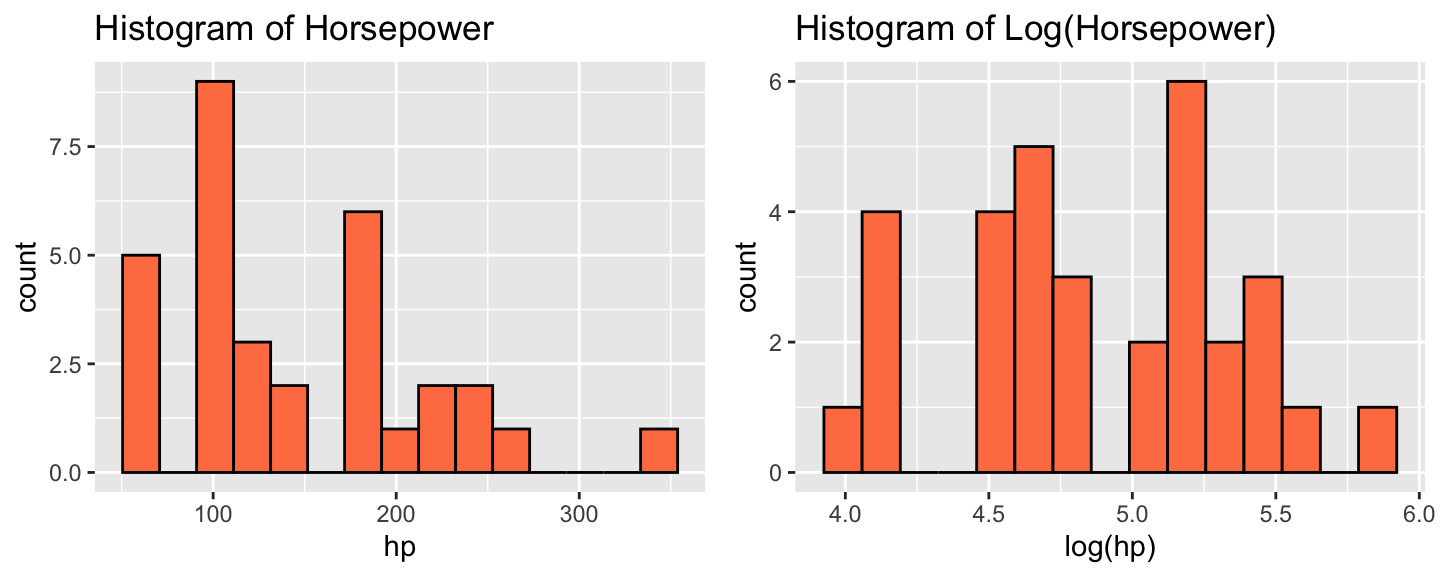## pairs() shows bivariate scatterplots

pairs(cars_subset %>% select(disp, hp, wt, drat), cex = 2)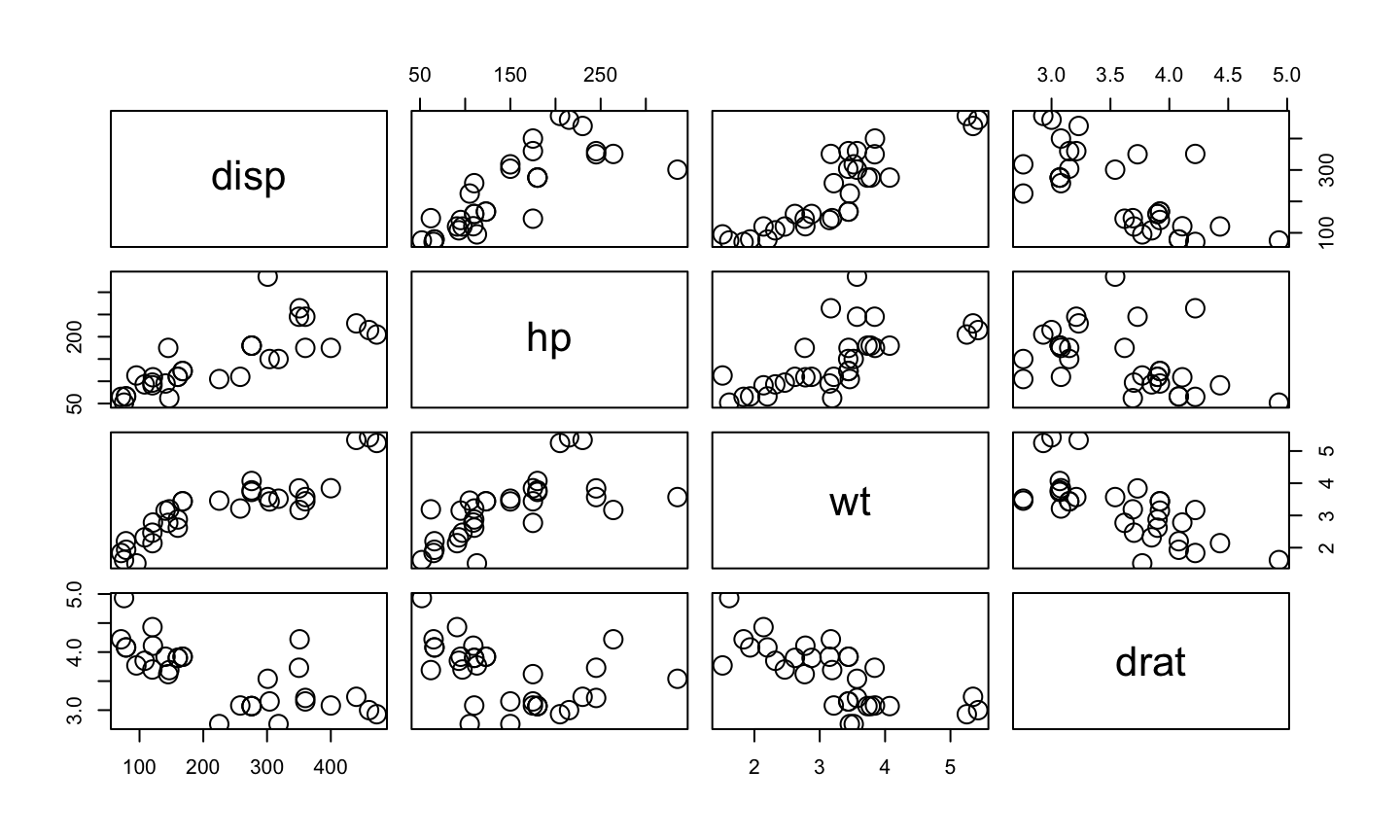## corrplot() returns pretty correlation diagrams

corrplot::corrplot.mixed(cor(cars_subset %>% select(disp, hp, wt, drat)))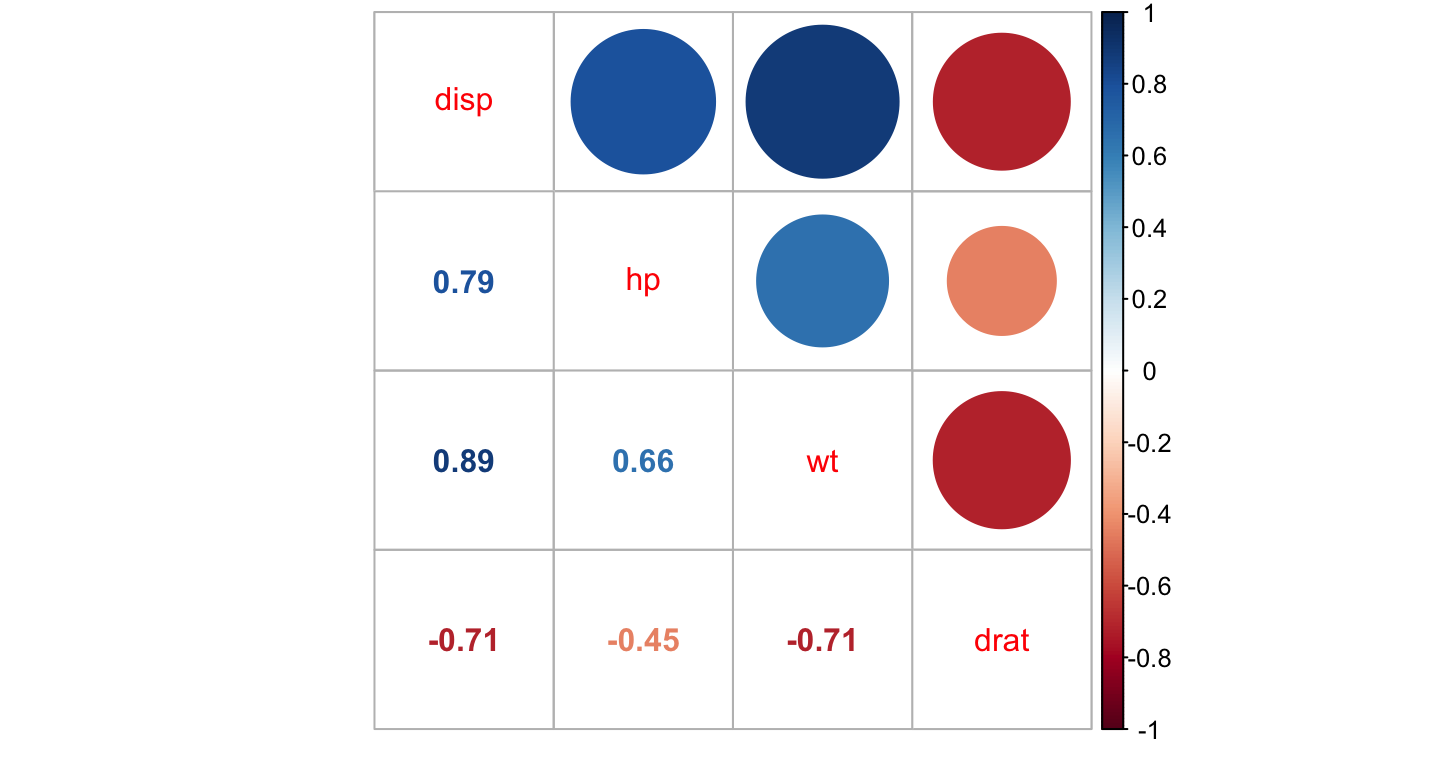## Variance inflation factor (VIF)

Variance inflation factor (VIF) can be used to determine how highly correlated predictors affect one another in regression.

Simply, if two predictors are highly correlated, it can be hard to determine which variable has an effect on a relationship. High VIF values make large uncertainty in the effects of a predictor.

diag(solve(cor(cars_subset %>% select(disp, hp, wt, drat))))
##     disp       hp       wt     drat
## 8.209402 2.894373 5.096601 2.279547

Here, a car’s horsepower, weight, and displacement are all correlated with one another. If gas mileage is poor, which one of these is the culprit?

## 4. Fitting Model Objects

Function calls in all major model-fitting packages take the form:

## lm(Response ~ Predictors)

By design, the intercept coefficient, $$\beta_0$$ is assumed to be added.

In a practical example:

## lm(mpg ~ hp, data = cars_subset)

Translates to: $Y = \beta_0 + \beta_1 * Horsepower + \epsilon$

## Model Notation

Removing the intercept

You’ll have to specify this manually with “0 +” or “-1”

coef(lm(mpg ~ hp, data = cars_subset))
## (Intercept)          hp
## 30.09886054 -0.06822828
coef(lm(mpg ~ 0 + hp, data = cars_subset))
##        hp
## 0.1011206

## Model Notation (Continued)

The “+” operator

coef(lm(mpg ~ hp + wt + disp + drat, data = cars_subset))
##  (Intercept)           hp           wt         disp         drat
## 29.148737553 -0.034783534 -3.479667528  0.003815241  1.768048769

## Model Notation (Continued)

Interaction terms

Interaction terms are designated through “:”

coef(lm(mpg ~ hp:wt, data = cars_subset))
## (Intercept)       hp:wt
## 27.74564216 -0.01487156

Including the "*" operator returns first-order terms and their interactions

coef(lm(mpg ~ hp*wt, data = cars_subset))
## (Intercept)          hp          wt       hp:wt
## 49.80842343 -0.12010209 -8.21662430  0.02784815

## Model Notation (Continued)

Removing Predictors

Sometimes, you may want to remove a predictor from a model formula with “-”

coef(lm(mpg ~ hp*wt - hp:wt, data = cars_subset))
## (Intercept)          hp          wt
## 37.22727012 -0.03177295 -3.87783074

## Model Notation (Continued)

Including all predictors with “.”

coef(lm(mpg ~ ., data = cars_subset))
##  (Intercept)         disp           hp           wt         drat
## 29.148737553  0.003815241 -0.034783534 -3.479667528  1.768048769

Note: Previous operations still apply!

coef(lm(mpg ~ . * ., data = cars_subset))
##   (Intercept)          disp            hp            wt          drat
##  7.058361e+01 -2.249685e-02 -1.146294e-01 -1.371510e+01 -4.748239e+00
##       disp:hp       disp:wt     disp:drat         hp:wt       hp:drat
##  1.594262e-04  8.669162e-04 -1.930254e-03  2.236928e-02 -7.905494e-03
##       wt:drat
##  1.747632e+00

## Model Notation (Continued)

Inhibiting interpretation

If you want to create new variables that are defined by predictors multiplied or added together, operations can be inhibited with “I()”

coef(lm(mpg ~ I(hp * wt), data = cars_subset))
## (Intercept)  I(hp * wt)
## 27.74564216 -0.01487156

## Model Notation (Continued)

Exponential Terms

“I()” is important for polynomials. Exponential terms are assigned through “^”. However, without I() this operation will produce first-and second-order terms!

coef(lm(mpg ~ hp + I(hp^2), data = cars_subset))
##   (Intercept)            hp       I(hp^2)
## 40.4091172029 -0.2133082599  0.0004208156
coef(lm(mpg ~ hp + hp^2, data = cars_subset))
## (Intercept)          hp
## 30.09886054 -0.06822828

## Model Notation (Continued)

“^” with multiple coefficients:

coef(lm(mpg ~ (hp + wt)^2, data = cars_subset))
## (Intercept)          hp          wt       hp:wt
## 49.80842343 -0.12010209 -8.21662430  0.02784815

## Quiz!

My data frame contains 5 variables: mpg, hp, wt, disp, drat

## lm(mpg ~ hp*wt*disp*drat - hp:wt:disp:drat, data = cars_subset)
## lm(mpg ~ .*.*. , data = cars_subset)
## lm(mpg ~ (hp+wt+disp+drat)^3, data = cars_subset)

What coefficients do these different models produce?

Trick question – they’re all the same!

coef(lm(mpg ~ disp * hp * wt * drat - hp:wt:disp:drat, data = cars_subset))
##   (Intercept)          disp            hp            wt          drat
##  5.951333e+01  9.172766e-01 -1.317022e+00 -2.669129e+01 -3.043506e+00
##       disp:hp       disp:wt         hp:wt     disp:drat       hp:drat
## -5.036538e-04 -2.105942e-01  4.567297e-01 -3.170942e-01  3.662498e-01
##       wt:drat    disp:hp:wt  disp:hp:drat  disp:wt:drat    hp:wt:drat
##  6.112672e+00 -1.062700e-04  3.137755e-04  7.293891e-02 -1.346007e-01

Trick question – they’re all the same!

coef(lm(mpg ~ .*.*. , data = cars_subset))
##   (Intercept)          disp            hp            wt          drat
##  5.951333e+01  9.172766e-01 -1.317022e+00 -2.669129e+01 -3.043506e+00
##       disp:hp       disp:wt     disp:drat         hp:wt       hp:drat
## -5.036538e-04 -2.105942e-01 -3.170942e-01  4.567297e-01  3.662498e-01
##       wt:drat    disp:hp:wt  disp:hp:drat  disp:wt:drat    hp:wt:drat
##  6.112672e+00 -1.062700e-04  3.137755e-04  7.293891e-02 -1.346007e-01

Trick question – they’re all the same!

coef(lm(mpg ~ (disp + hp + wt + drat)^3, data = cars_subset))
##   (Intercept)          disp            hp            wt          drat
##  5.951333e+01  9.172766e-01 -1.317022e+00 -2.669129e+01 -3.043506e+00
##       disp:hp       disp:wt     disp:drat         hp:wt       hp:drat
## -5.036538e-04 -2.105942e-01 -3.170942e-01  4.567297e-01  3.662498e-01
##       wt:drat    disp:hp:wt  disp:hp:drat  disp:wt:drat    hp:wt:drat
##  6.112672e+00 -1.062700e-04  3.137755e-04  7.293891e-02 -1.346007e-01

All first, second, and third order terms

## A guide to your fitted model object

Fitting a new model

mymodel <- lm(mpg ~ hp, data = cars_subset)

What formula did I run?

mymodel\$call
## lm(formula = mpg ~ hp, data = cars_subset)
formula(mymodel)
## mpg ~ hp

## Your fitted model object (continued)

summary() provides a general overview of your model

##
## Call:
## lm(formula = mpg ~ hp, data = cars_subset)
##
## Residuals:
##     Min      1Q  Median      3Q     Max
## -5.7121 -2.1122 -0.8854  1.5819  8.2360
##
## Coefficients:
##             Estimate Std. Error t value Pr(>|t|)
## (Intercept) 30.09886    1.63392  18.421  < 2e-16 ***
## hp          -0.06823    0.01012  -6.742 1.79e-07 ***
## ---
## Signif. codes:  0 '***' 0.001 '**' 0.01 '*' 0.05 '.' 0.1 ' ' 1
##
## Residual standard error: 3.863 on 30 degrees of freedom
## Multiple R-squared:  0.6024, Adjusted R-squared:  0.5892
## F-statistic: 45.46 on 1 and 30 DF,  p-value: 1.788e-07

## Your fitted model object (continued)

anova() provides the sum-of-squares decomposition

## Analysis of Variance Table
##
## Response: mpg
##           Df Sum Sq Mean Sq F value    Pr(>F)
## hp         1 678.37  678.37   45.46 1.788e-07 ***
## Residuals 30 447.67   14.92
## ---
## Signif. codes:  0 '***' 0.001 '**' 0.01 '*' 0.05 '.' 0.1 ' ' 1

## Your fitted model object (continued)

Add new predictors to an existing model with update()

update(mymodel, formula = ~ . + disp)
##
## Call:
## lm(formula = mpg ~ hp + disp, data = cars_subset)
##
## Coefficients:
## (Intercept)           hp         disp
##    30.73590     -0.02484     -0.03035

## 5. Regression Diagnostics

There are many useful links to interpret diagnostic plots. Here, I’ll focus on diagnostics for a simple (normal) linear model.

Plot 1 – Residuals vs. Fitted

Shows the relationship between estimated response (Fitted values) and error (Residuals). Ideally, these fall along a straight line with even spread.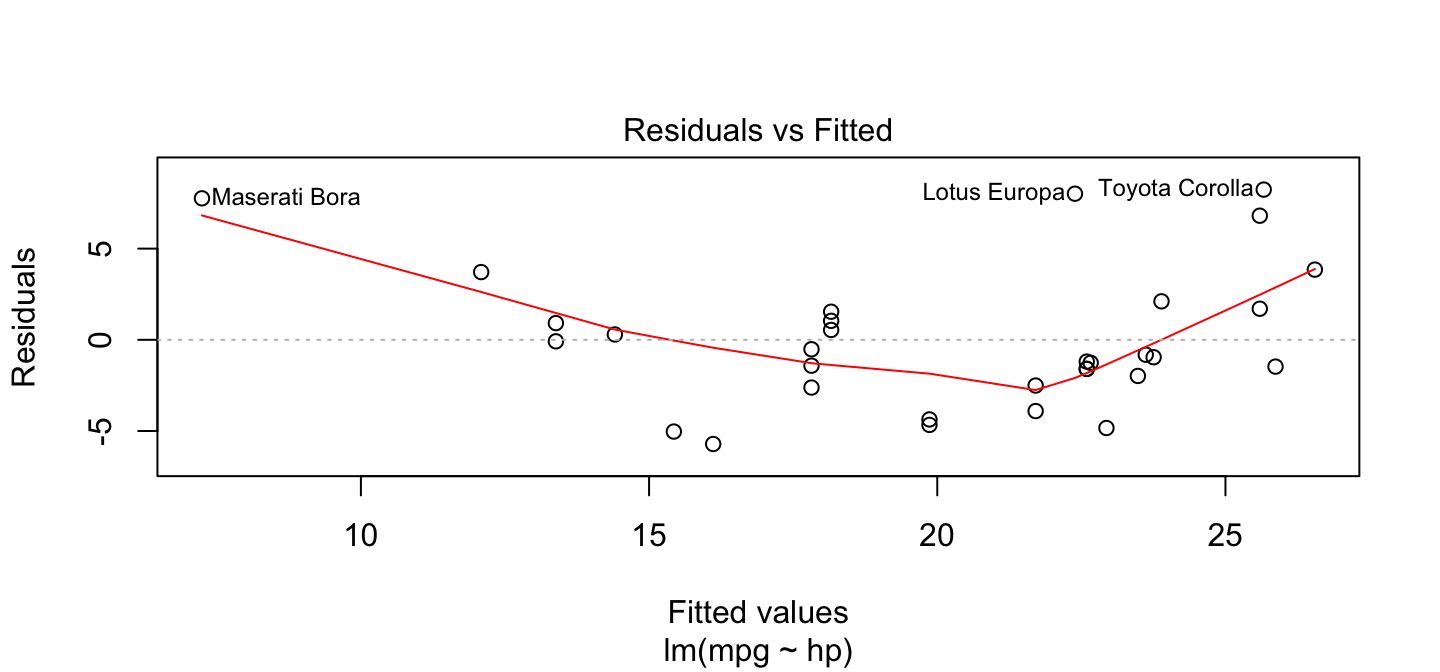## Regression Diagnostics

Plot 2 – Quantile-Quantile (QQ) Plot

The QQ plot demonstrates how closely our observed residuals resemble a normal distribution. Values should fall along the 1:1 line.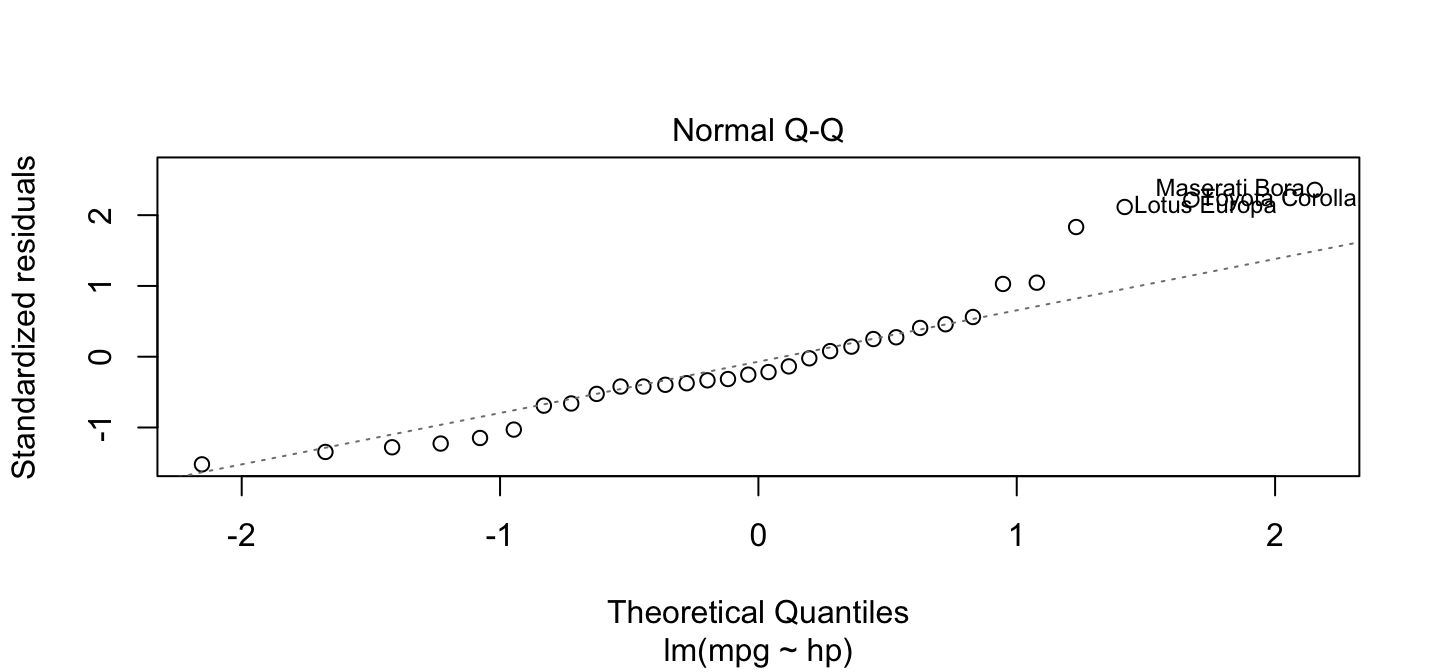## Regression Diagnostics

Plot 3 – Scale-Location

Another way to see non-random patterns in residuals. Trends with sloped lines show greater variance as predicted values increase (similar to a funnel-shaped residuals vs. fitted plot)